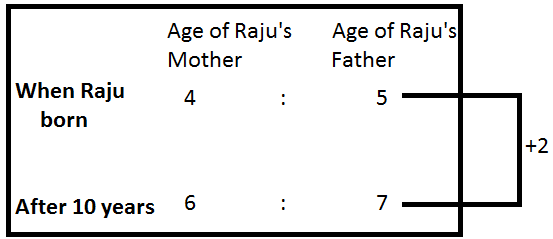Question of The Day08-10-2020

At the time of Raju’s birth, the ratio of ages of his mother and father was 4 : 5. On his tenth birthday, the ratio of the ages of his mother and father changes to 6 : 7. If Raju’s mother and father were married 2 years before his birth, then what will be the sum of ages of Raju’s mother and Raju’s father at the time of their marriage?

Correct Answer : c ) 41 years

Explanation :

Quick Approach

The difference between the ages of two persons will always remain the same.Thus, from the above figure

2 units = 10 years

⇒ 1 unit = 5 years

Sum of ages of Raju’s mother and Raju’s Father at the time of Raju’s birth = 4 + 5 = 9 units = 9 * 5 = 45 years

Sum of ages of Raju’s mother and Raju’s father at the time of their marriage = 45 – 2 – 2 = 41 years

Hence, (c) is the correct answer.

Basic Approach

According to the question,

At the time of Raju’s birth, the ratio of his mother and father was 4 : 5

Let us assume the age of Raju’s mother at the time of Raju’s birth is 4x years

So, the age of Raju’s father at the time of Raju’s birth is 5x

According to the question, after 10 years their age ratio becomes 6 : 7

So,

$${4x+10 \over 5x+10}={6 \over 7}$$

⇒ 28x + 70 = 30x + 60

⇒ 2x = 10

⇒ x = 5 years

So, age of Raju’s mother at the time of Raju’s birth = 4x = 20 years

And, age of Raju’s father at the time of Raju’s birth = 5x = 25 years

Sum of ages of Raju’s mother and Raju’s father at the time of their marriage = 20 – 2 + 25 – 2 = 41 years.

Hence, (c) is the correct answer.

Such type of question is asked in various government exams like RRB JE, RRB NTPC, RRB GROUP D, RRB OFFICER SCALE-I, IBPS PO, IBPS SO, RRB Office Assistant, IBPS Clerk, RBI Assistant, IBPS RRB OFFICER SCALE 2&3, UPSC CDS, UPSC NDA, UP SI etc.

Read Daily Current Affairs, Banking Awareness, Hindi Current Affairs, Word of the Day, and attempt free mock tests at PendulumEdu and boost your preparation for the actual exam.0